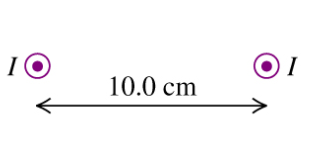# Problem: Two long, straight, parallel wires. 10.0 cm apart carry equal 4.00-A currents in the same direction. as shown in the figureFind the magnitude of the magnetic field at point P3, 20.0 cm directly above P1.What is its direction?A) to the left B) to the right C) upward D) downwardE) no field

###### FREE Expert Solution

Magnetic field:

$\overline{){\mathbf{B}}{\mathbf{=}}\frac{{\mathbf{\mu }}_{\mathbf{0}}\mathbf{i}}{\mathbf{2}\mathbf{\pi }\mathbf{r}}}$

r = sqrt [202 + (10/2)2] = 20.6 cm

Magnetic field due to the first wire:

B1 = (4π × 10-7)(4.00)/(2π)(20.6 × 10-2) = 3.88 × 10-6 T

Magnetic field due to the second wire:

B2 = (4π × 10-7)(4.00)/(2π)(20.6 × 10-2) = 3.88 × 10-6 T

The angle between B1 and B2 is θ

82% (182 ratings)###### Problem Details

Two long, straight, parallel wires. 10.0 cm apart carry equal 4.00-A currents in the same direction. as shown in the figureFind the magnitude of the magnetic field at point P3, 20.0 cm directly above P1.

What is its direction?

A) to the left

B) to the right

C) upward

D) downward

E) no field